## Important topics covered in Force and Pressure notes:

• Pre Knowledge
• Force
• Effect of force
• Contact force
• Non-contact forces
• Magnetic forces
• Electrostatic forces
• Gravitational force
• Pressure
• Pressure Exerted by Liquids and Gases
• Atmospheric Pressure

• Different actions which we perform in our day to day life are playing, lifting weight, pushing, picking, pulling etc. creates change in position of the object.
• We observe different types of motion in object after these task.

## Force:• Push or pull on any object is said to be force.
• Push: When an object moves away from the applier of force, the object is said to be pushed.
• Pull: When object moves towards the direction of application of force than object is said to be pulled.
• Simply, we can define force as the physical cause which either changes or try to change the position, shape, size of any object.
• The interaction of one objects with the other also leads to the force between them.
• The magnitude and direction of force decides the effect of force.

### Effect and application of force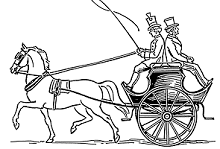• When force is applied in the direction of motion of body then it adds up to the actual force.
• When force is applied in opposite direction of motion of a body then net force is the difference of the two forces.
• Force can cause a body to move from rest.
• A moving body can be brought to rest after the application of force.
• Application of force can change the direction of moving body.
• It can change the speed of a moving body.
• It can also change the shape and size of body.

## Contact forces

Those forces which cause its effect by making contact with the object is said to be contact force. Some contact forces are given below. Take a look at it:

### Muscular force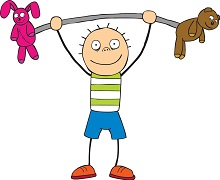• Force which involves the action of muscles.
• Human, as well as animals, use muscular force to perform various tasks.
• By muscular force, a bullock is able to pull a cart.

### Friction• To oppose the relative motion between two surfaces, when one surface is moving over the other a force which acts is said to be friction.
• Friction always opposes motion because it acts in opposite direction to motion.
• There would be no friction if there is no contact between two surfaces.
• Frictional force also depends upon the nature of the contact surface.

## Non-contact force:

The force which can cause effect without making a contact with object is said to be non-contact forces. Let’s take a look at the different types of non-contact forces.

### Magnetic Force: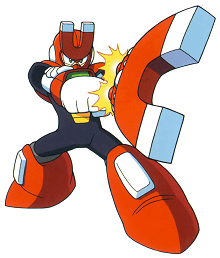• The force applied by a magnet on any magnetic object is said to be magnetic force.
• A magnet can exert its force on an object even from a distance and does not need to touch that object.
• Iron, steel, cobalt etc. are some magnetic objects.
• The magnetic force may be attractive or repulsive.

### Electrostatic force: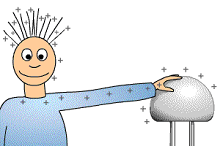• The force which is produced due to electric charges is called electrostatic forces.
• This force exerted by a charged body on another charged or uncharged body
• Electrostatic force can also be attractive or repulsive.

### Gravitational force:• Every object in the universe exerts a force on another object and this force is called gravitational force.
• The celestial bodies keep their defined position in the space due to the gravitational force of celestial bodies.
• It is attractive in nature and cause due to mass of the body.
• Any object, when thrown upwards, falls down to the earth’s surface due to gravitational force.

## Pressure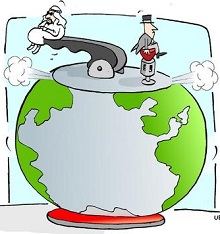• Force acting per unit area is called pressure.
• Pressure = $$\frac{force}{Surface\:Area\:on\:which\:it\:acts}$$
• The unit of pressure is N/m2. We can also call it Pascal.
• Pressure is directly proportional to force.
• Area of surface is inversely proportional to pressure. So smaller the surface, higher will be its pressure.
• Liquids exert a force on the wall of container and effect of pressure increases with depth.
• At same depth in the container, the pressure is the same.
• Gases also exert pressure on the walls of their container.

## Fluid: Pressure Exerted by Liquid and Gases

• Those substances which can flow is said to be fluid.
• Fluids include liquids as well as gases.
• At same depth, fluid exerts equal pressure.

## Atmospheric Pressure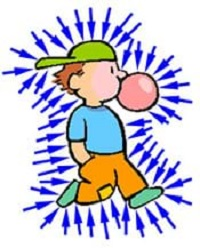• This envelope of air surrounding the earth is known as the atmosphere.
• Atmospheric air extends up to many km’s above the earth’s surface.
• The pressure exerted by the air on any surface is known as atmospheric pressure.

## FAQ on Force and Pressure

### What do you mean by force and pressure?

Force is the physical cause which either changes or try to change the position, shape, size of any object. Pressure is the force applied to any object per unit area. Or simply, the ratio of applied force to the area on which it is applied gives pressure.

### How is pressure related to force and area explain with an example?

Pressure is the ratio of force applied to the area on which it is applied. So we can say that pressure on any object is directly proportional to the Force applied on that object whereas inversely proportional to the area on which it is applied. Smaller the area greater will be the pressure.

### How is pressure related to force?

Pressure is directly proportional to the applied force. As the force on an object increases, the pressure also increases. This is derived from the formula of pressure according to which the ratio of applied force and area of the application gives the pressure on any object.

### What are the effects of force?

Effects of force are:

• Change in position
• Change in shape, size
• Increase/decrease speed
• Change in direction of moving object
• Accelerate the body
• Retardates the body

### What are the 4 types of force?

There are basically four types of natural forces:

• Electrostatic force
• Magnetic force
• Gravitational force
• Frictional force

### What are the factors on which the pressure at a point in a liquid depends?

The factors on which pressure at a point in a liquid is:

• Depth of the position below the surface (h)
• Density of the liquid (d)
• Acceleration due to gravity (g)